# 机器学习基石笔记(2)

## learning的两个问题

• 能否保证$E_{in} \thickapprox E_{out}$
• 能否保证$E_{in} \thickapprox 0$

## M的实际有效值

### PAL感知机

$P[ | E_{in} - E_{out} | > \epsilon ] \leq 2 \cdot M \cdot exp \lgroup -2 \epsilon^2N\rgroup$ 从式子中，可以看出当M比较小的时候，即假设空间$\mathcal{H}$中可以选择的函数比较少，这样就很容易保证坏数据出现的概率很小，但是因为选择性太少了，我们无法保证$E_{in}$尽可能小。但是M比较大的时候，选择多了， 但是却让坏数据概率大了。 现在，我们考虑一下， BAD DATA的推导公式: $P[ BAD | \mathcal{H}] \leq_{union bound} P(BAD | h_1) + P(BAD | h_2) + ... + P(BAD | h_M)$ 这里面有个问题，在计算右边时，直接对每一个$h$求和，这个是上界，也就是当每一项都独立时，该等号才成立，但是实际情况下，并非这样，比如$h_1, h_2$两个很相似的$hypothsis$, 也就是说，满足如下两个要求: - $E_{out}(h_1) \thickapprox E_{out}(h_2)$ - $E_{in}(h_1) \thickapprox E_{in}(h_2)$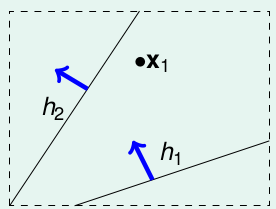learning

• $h_1-like(x_1) = o$$h_1$类似，将$x_1$归为 o
• $h_2-like(x_2) = x$$h_1$类似，将$x_1$归为 x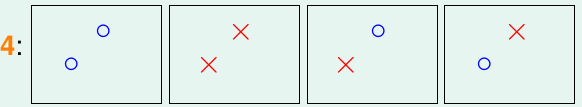learning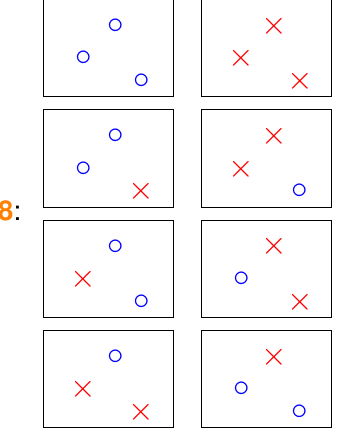learning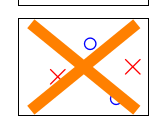learning

### Growth Funciton 成长函数

N $m_{\mathcal{H}}(N)$
1 2
2 4
3 max(6,8) = 8
4 14 < $2^4$

1.Positive Rays: $h(x) = sign(x-a)$, 输入空间为一维向量， 当$x_i \geq a$, 输出 +1, 当$x_i < a$ 时， 输出 -1. 如下: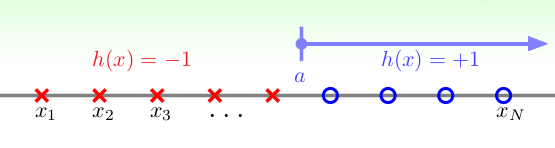可以发现，对于N个的输入，一共可以产生N+1个dichotomies, 就相当在N个位置中找一个位置作为a,然后加上一个边界，所以一共有N+1种.即: $m_{\mathcal{H}}(N) = N + 1$

2.Positive Intervals: 其实就是上一个的双向版， 输入空间仍为一维向量，当 $x_i \in [l ,r] , y_i = 1; x_i \in [l, r], y_i = -1$, 如下: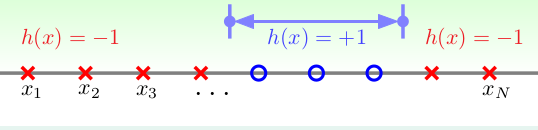3.Convex Sets: 凸集合，如下图，其中 $R^2$, 当 $x_i$在凸形内，$h(x_i) = +1$, 反之则$h(x_i) = -1$, 如下: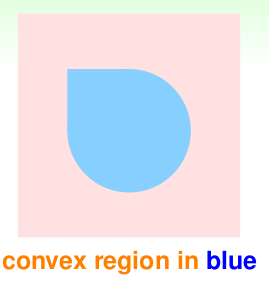## break point & shatter

• $m_{\mathcal{H}}(N) = N+1$, Postive Rays
• $m_{\mathcal{H}}(N) = \frac{1}{2}N_2 + \frac{1}{2}N+1$,Postive Inteervals
• $m_{\mathcal{H}}(N) = 2^N$,convex sets
• $m_{\mathcal{H}}(N) = 未知,\ 2D\ perceptron.\ m_{\mathcal{H}}(4) = 14 < 2^4$

• postive rays: $m_{}(N) = N + 1 = O(N), break-point: 2$
• postive intervals: $m_{\mathcal{H}}(N) = \frac{1}{2}N_2 + \frac{1}{2}N+1=O(N^2),\ break-point: 3$
• convex sets: $m_{}(N) = 2^N, no break-point$
• 2D perceptrons: $break-point : 4$

## 总结

$P[ | E_{in} - E_{out} | > \epsilon ] \leq 2 \cdot effective(N) \cdot exp \lgroup -2 \epsilon^2N\rgroup$